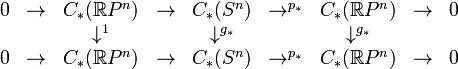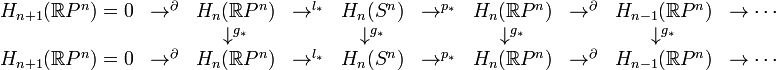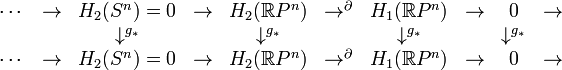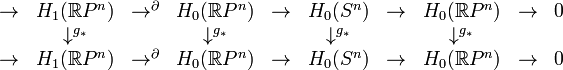# 0708-1300/Class notes for Tuesday, April 3

Announcements go here

INCOMPLETE - Will be finished (most likely) Tomorrow

## Typed Notes

The notes below are by the students and for the students. Hopefully they are useful, but they come with no guarantee of any kind.

### First Hour

Theorem 1: Borsuk-Ulam

f:S^n\rightarrow\mathbb{R}^n has a point x\in S^n so f(x) = f(-x) \Leftrightarrow there does not exists a g:S^n\rightarrow S^{n-1} that is odd, i.e., g(-x)=-g(x)

(\Leftarrow) If an f with no such fixed point existed then g(x) = (f(x) - f(-x))/||f(x) - f(-x)||

Theorem 2:

If g:S^n\rightarrow S^n is odd then g_*:H_n(S^n,\mathbb{Z}/2)\rightarrow H_n(S^n,\mathbb{Z}/2), ie g_*:\mathbb{Z}/2\rightarrow\mathbb{Z}/2, is the identity.

Claim:

Theorem 2 implies Borsuk-Ulam.

Indeed, if g:S^n\rightarrow S^{n-1} is odd and exists then S^n\rightarrow^g S^{n-1}\hookrightarrow\S^n where the final map is the inclusion to the equation then the composition of these maps \tilde{g}:S^n\rightarrow S^n is odd. But, \tilde{g} lives on the equator only and so is homotopic to a constant. Hence, \tilde{g}_* = (const)_* = 0. This establishes the contradiction.

Q.E.D

Proof of Theorem 2

We use the technique of a "transfer sequence" over \mathbb{Z}/2

Let p:X\rightarrow B be a two sheeted covering

Over \mathbb{Z}/2 we have the following short exact sequence:

\rightarrow C_*(B)\rightarrow^{l_*}C_*(X)\rightarrow^{p_*}C_*(B)\rightarrow 0

where l is the sum of two liftings from the base.

The above induces a long exact sequence of homology groups.$\begin{matrix} 0&\rightarrow&C_*(\mathbb{R}P^n)&\rightarrow & C_*(S^n)&\rightarrow^{p_*} & C_*(\mathbb{R}P^n)&\rightarrow&0\\ &&\downarrow^1&&\downarrow^{g_*}&&\downarrow^{g_*}&&\\ 0&\rightarrow&C_*(\mathbb{R}P^n)&\rightarrow & C_*(S^n)&\rightarrow^{p_*} & C_*(\mathbb{R}P^n)&\rightarrow&0\\ \end{matrix}$

The right square commutes irregardless of where g is even or odd and the left square commutes if the g is odd.

We get the following (very) long exact sequence:$\begin{matrix} H_{n+1}(\mathbb{R}P^n) = 0 & \rightarrow^{\partial}& H_n(\mathbb{R}P^n) & \rightarrow^{l_*} & H_n(S^n) & \rightarrow^{p_*} & H_n(\mathbb{R}P^n)& \rightarrow^{\partial}& H_{n-1}(\mathbb{R}P^n) & \rightarrow \cdots\\ &&\downarrow^{g_*}&&\downarrow^{g_*}&&\downarrow^{g_*}&&\downarrow^{g_*}&\\ H_{n+1}(\mathbb{R}P^n) = 0 & \rightarrow^{\partial}&H_n(\mathbb{R}P^n)&\rightarrow^{l_*} & H_n(S^n) & \rightarrow^{p_*} & H_n(\mathbb{R}P^n)&\rightarrow^{\partial}&H_{n-1}(\mathbb{R}P^n)&\rightarrow \cdots\\ \end{matrix}$$\begin{matrix} \cdots & \rightarrow & H_{2}(S^n) = 0 & \rightarrow & H_2(\mathbb{R}P^n) & \rightarrow^{\partial} & H_1(\mathbb{R}P^n) & \rightarrow & 0 & \rightarrow \\ &&\downarrow^{g_*}&&\downarrow^{g_*}&&\downarrow^{g_*}&&\downarrow^{g_*}&\\ \cdots & \rightarrow & H_{2}(S^n) = 0 & \rightarrow & H_2(\mathbb{R}P^n) & \rightarrow^{\partial} & H_1(\mathbb{R}P^n) & \rightarrow & 0 & \rightarrow \\ \end{matrix}$$\begin{matrix} \rightarrow & H_1(\mathbb{R}P^n)& \rightarrow^{\partial} & H_0(\mathbb{R}P^n)& \rightarrow & H_0(S^n) & \rightarrow & H_0(\mathbb{R}P^n) & \rightarrow & 0 \\ &\downarrow^{g_*}&&\downarrow^{g_*}&&\downarrow^{g_*}&&\downarrow^{g_*}&&\\ \rightarrow & H_1(\mathbb{R}P^n)& \rightarrow^{\partial} & H_0(\mathbb{R}P^n)& \rightarrow & H_0(S^n) & \rightarrow & H_0(\mathbb{R}P^n) & \rightarrow & 0 \\ \end{matrix}$

The above three diagrams are meant to be connected end to end.

It is difficult to write, but fairly easy to see, that by starting at the far right (the right of the bottom most diagram) and working left that:

H_k (\mathbb{R}P^n,\mathbb{Z}/2) = \mathbb{Z}/2 \forall p<n

and that g_* is in fact the identity at all places. Q.E.D